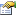﻿ CodecsVectorImageInfo Class Properties | Leadtools.Codecs | Raster, Medical, Document Help
←Select platform
In This Topic ▼

# CodecsVectorImageInfo Class Properties

Summary

For a list of all members of this type, see CodecsVectorImageInfo members

Public Properties

Name DescriptionIsVectorFile Gets a value indicating whether the image file or stream is a vector image format.ParallelogramMaxX The maximum point of the bounding parallelogram of the vector fileParallelogramMaxY The maximum point of the bounding parallelogram of the vector fileParallelogramMaxZ The maximum point of the bounding parallelogram of the vector fileParallelogramMinX The minimum point of the bounding parallelogram of the vector fileParallelogramMinY The minimum point of the bounding parallelogram of the vector fileParallelogramMinZ The minimum point of the bounding parallelogram of the vector fileUnit Gets the unit of measure for the vector image file.